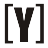How to Convert Hexadecimal to Decimal: insert number in Hex box and press convert button to get result in Decimal.

16
10
10
2
Decimal to Hex Converter

## What is Decimal number?

A decimal number is a number expressed in the base-10 positional numbering system, which uses ten digits (0-9) to represent any numerical quantity. Each digit in a decimal number represents a specific value that is determined by its position relative to the decimal point.

The digit to the left of the decimal point represents the whole number value, while the digits to the right of the decimal point represent fractional parts of the number.

## How to convert Hex to Decimal?

1. Write down the hex number.
2. Assign a decimal value to each hex digit, using the following table:

Hex digit Decimal value
0 0
1 1
2 2
3 3
4 4
5 5
6 6
7 7
8 8
9 9
A 10
B 11
C 12
D 13
E 14
F 15
3. Multiply each digit by its corresponding decimal value, starting from the rightmost digit and working left.
4. Add up the products to get the decimal equivalent of the hex number.

Examples:
Question: Convert hex number “1A” to decimal?

1. Write down “1A”
2. Assign decimal values: 1=1, A=10
3. Multiply each digit by its value: 1 x 16^1 + 10 x 16^0 = 26
4. The decimal equivalent of “1A” is 26.

So, “1A” in hexadecimal is equivalent to the decimal number 26.

### Hex to Decimal Conversion Table for the digits 0 to F

Hex digit Decimal value
0 0
1 1
2 2
3 3
4 4
5 5
6 6
7 7
8 8
9 9
A 10
B 11
C 12
D 13
E 14
F 15

This table can be used to convert any hexadecimal number to its decimal equivalent by multiplying each digit by its corresponding decimal value and adding up the products.YoosFuhl.com Would you like to receive notifications on latest updates? No Yes